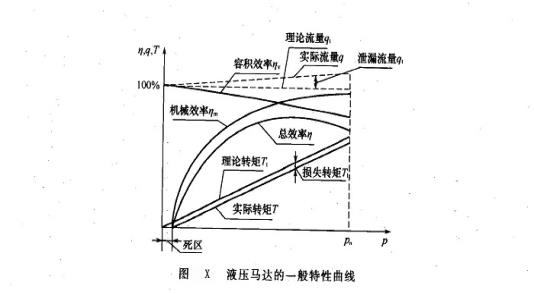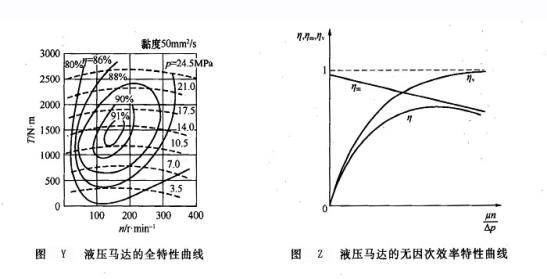Created on：2021-06-11 10:27

# Practical calculation formula and characteristic curve

Practical calculation formula and characteristic curve

1.7.2 engineering practical calculation formula

In order to facilitate the selection, use and maintenance of hydraulic motor, the following table gives the calculation formula of hydraulic motor commonly used in engineering.

Calculation formula of hydraulic motor commonly used by engineering companies

 project Calculation formula Symbolic meaning name Company Theoretical flow QT L/min Qt=Vn/1000 V -- displacement of hydraulic motor, ml / R; N -- speed of hydraulic motor, R / min; Δ P -- pressure difference between inlet and outlet of hydraulic motor, MPa Actual flow Q Q=qt/ηv=Vn/(1000vη) Output power Po kW Po=△pqη/60 Input power pi Pi=△pq/60 Theoretical torque TT N·m Tt=△pV/(2π) Actual torque T T=△pVηm/(2π) Volumetric efficiency η V % ηv=qt/q Mechanical efficiency η M ηm=T/Tt 总效率η η=ηvηm

1.7.3 characteristic curve

The characteristic curve of hydraulic motor also includes general characteristic curve, full characteristic curve and dimensionless characteristic curve. Understanding these characteristic curves is beneficial to the characteristic analysis, development, use and maintenance of hydraulic motor.

(1) General characteristic curve the general characteristic curve of hydraulic motor is the relationship curve between performance parameters such as efficiency, flow, torque and working pressure P, as shown in Figure X. As can be seen from the figure:

① These parameters of hydraulic pump are different under different working pressure.

② The actual flow rate Q increases with the increase of working pressure P, but the volumetric efficiency η decreases with the increase of working pressure P. Due to the existence of internal friction, the working pressure P is near zero, which makes the motor torque, mechanical efficiency and total efficiency characteristic curve have a "dead zone", that is, the actual torque, mechanical efficiency and total efficiency are all zero. Only when the working pressure makes the motor reach the starting torque, it will start to run and output torque. The mechanical efficiency of the motor is η. Starting from zero, it increases rapidly with the increase of pressure, and then slows down, so the total efficiency starts from zero, and there is a highest point. Obviously, the hydraulic motor should work near this point.(2) In order to fully reflect the efficiency characteristics of hydraulic motor and facilitate users to understand and select the high efficiency working area, it is necessary to draw the characteristic curve within the whole speed and pressure range, which is called full characteristic curve (or full efficiency characteristic curve or general characteristic curve). As shown in figure y, the abscissa of the full characteristic curve is usually represented by speed n, and the ordinate represents torque T on one side and pressure on the other. The isoefficiency curve should be drawn in the graph.(3) Dimensionless characteristic curve the relationship between the basic characteristic parameters of a motor and the dimensionless variable (μ n / AP) (△ P, μ, n are the pressure difference, dynamic viscosity and speed of the motor respectively) is called the dimensionless characteristic curve of a hydraulic motor, which can represent numerous characteristic curves.

The dimensionless efficiency characteristic curve of hydraulic motor is shown in Figure Z. It can be seen that the volumetric efficiency η of hydraulic motor decreases with the increase of pressure difference △ P, increases with the increase of oil viscosity μ and speed n, and the mechanical efficiency η. The total efficiency η is also a function of μ n / AP.

Home    Article    Practical calculation formula and characteristic curve